PHY_10_34_V2_P_Charges in E
Оценка 4.8

# PHY_10_34_V2_P_Charges in E

Оценка 4.8
pptx
07.05.2020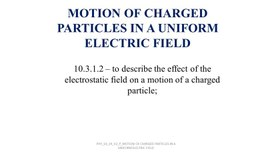PHY_10_34_V2_P_Charges in E.pptx

MOTION OF CHARGED PARTICLES IN A UNIFORM ELECTRIC FIELD

10.3.1.2 – to describe the effect of the electrostatic field on a motion of a charged particle;

PHY_10_34_V2_P_MOTION OF CHARGED PARTICLES IN A UNIFORM ELECTRIC FIELD

Review: What is a uniform electric field?

DRAW
a uniform electric field lines between two parallel plates that are d distance away from each other.

PHY_10_34_V2_P_MOTION OF CHARGED PARTICLES IN A UNIFORM ELECTRIC FIELD

Review: What is a uniform electric field?

PHY_10_34_V2_P_MOTION OF CHARGED PARTICLES IN A UNIFORM ELECTRIC FIELD

## When a particle of charge q and mass m is placed in an electric field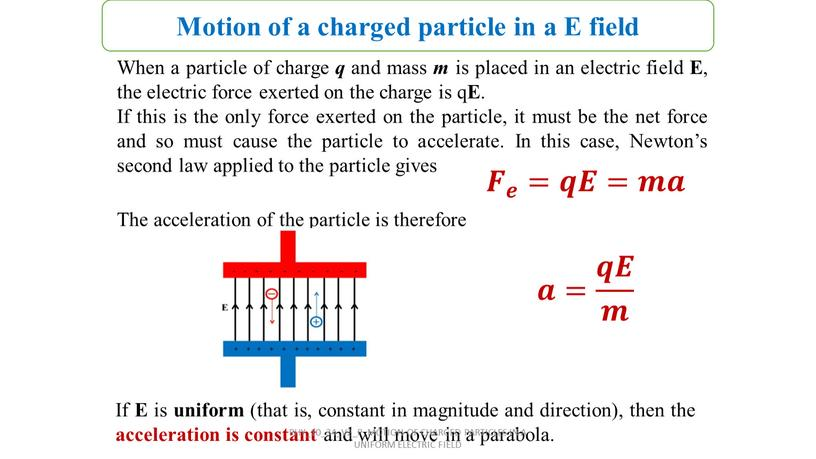When a particle of charge q and mass m is placed in an electric field E, the electric force exerted on the charge is qE.
If this is the only force exerted on the particle, it must be the net force and so must cause the particle to accelerate. In this case, Newton’s second law applied to the particle gives

𝑭 𝒆 =𝒒𝑬=𝒎𝒂

The acceleration of the particle is therefore

𝒂= 𝒒𝑬 𝒎

If E is uniform (that is, constant in magnitude and direction), then the acceleration is constant and will move in a parabola.

Motion of a charged particle in a E field

PHY_10_34_V2_P_MOTION OF CHARGED PARTICLES IN A UNIFORM ELECTRIC FIELD

## If the particle has a positive charge, then its acceleration is in the direction of the electric field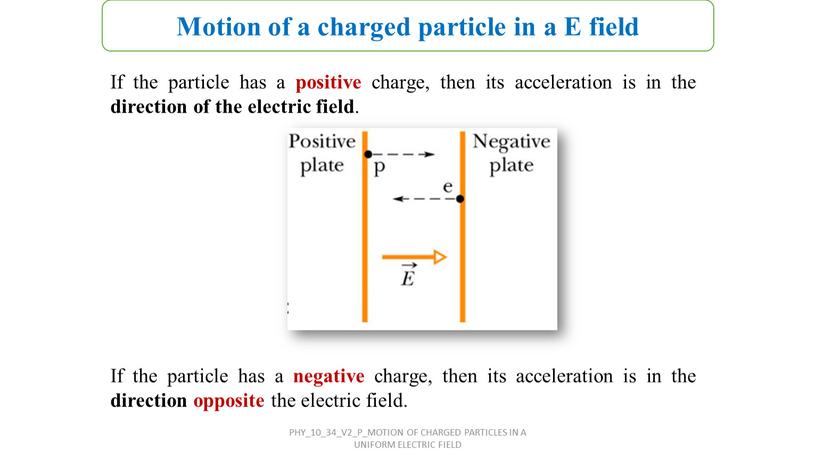If the particle has a positive charge, then its acceleration is in the direction of the electric field.

If the particle has a negative charge, then its acceleration is in the direction opposite the electric field.

Motion of a charged particle in a E field

PHY_10_34_V2_P_MOTION OF CHARGED PARTICLES IN A UNIFORM ELECTRIC FIELD

## Problem Solving An electron is placed in an uniform electric field of 4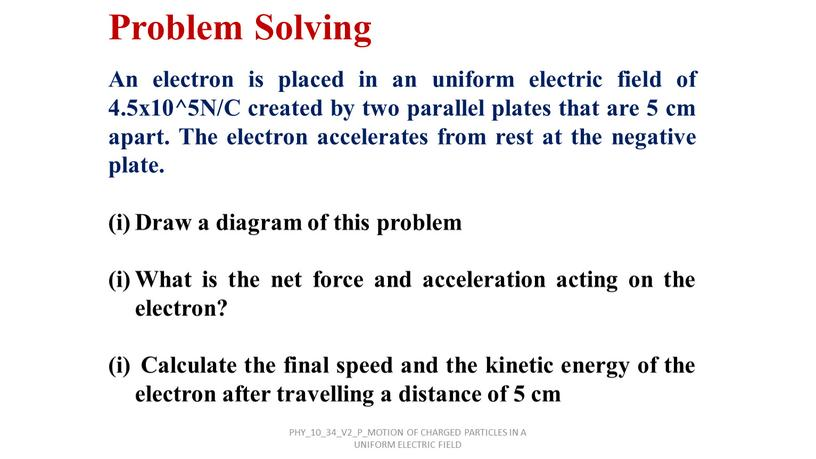Problem Solving

An electron is placed in an uniform electric field of 4.5x10^5N/C created by two parallel plates that are 5 cm apart. The electron accelerates from rest at the negative plate.

Draw a diagram of this problem

What is the net force and acceleration acting on the electron?

Calculate the final speed and the kinetic energy of the electron after travelling a distance of 5 cm

PHY_10_34_V2_P_MOTION OF CHARGED PARTICLES IN A UNIFORM ELECTRIC FIELD

## ANSWER: (i) (ii) 𝑭 𝒆 =𝒒𝑬=𝒆𝑬=𝟕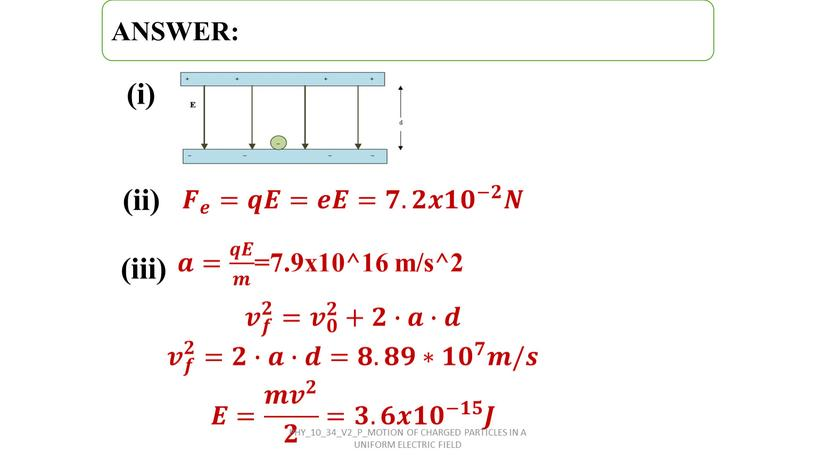(i)

(ii)

𝑭 𝒆 =𝒒𝑬=𝒆𝑬=𝟕.𝟐𝒙𝟏 𝟎 −𝟐 𝑵

(iii)

𝒂𝒂= 𝒒𝑬 𝒎 𝒒𝒒𝑬𝑬 𝒒𝑬 𝒎 𝒎𝒎 𝒒𝑬 𝒎 =7.9x10^16 m/s^2

𝒗 𝒇 𝟐 𝒗𝒗 𝒗 𝒇 𝟐 𝒇𝒇 𝒗 𝒇 𝟐 𝟐𝟐 𝒗 𝒇 𝟐 = 𝒗 𝟎 𝟐 𝒗𝒗 𝒗 𝟎 𝟐 𝟎𝟎 𝒗 𝟎 𝟐 𝟐𝟐 𝒗 𝟎 𝟐 +𝟐𝟐∙𝒂𝒂∙𝒅𝒅
𝒗 𝒇 𝟐 𝒗𝒗 𝒗 𝒇 𝟐 𝒇𝒇 𝒗 𝒇 𝟐 𝟐𝟐 𝒗 𝒇 𝟐 =𝟐𝟐∙𝒂𝒂∙𝒅𝒅=𝟖𝟖.𝟖𝟖𝟗𝟗∗ 𝟏𝟎 𝟕 𝟏𝟏𝟎𝟎 𝟏𝟎 𝟕 𝟕𝟕 𝟏𝟎 𝟕 𝒎𝒎/𝒔𝒔
𝑬= 𝒎 𝒗 𝟐 𝟐 =𝟑.𝟔𝒙 𝟏𝟎 −𝟏𝟓 𝑱

PHY_10_34_V2_P_MOTION OF CHARGED PARTICLES IN A UNIFORM ELECTRIC FIELD

Problem #2

PHY_10_34_V2_P_MOTION OF CHARGED PARTICLES IN A UNIFORM ELECTRIC FIELD

PHY_10_34_V2_P_MOTION OF CHARGED PARTICLES IN A UNIFORM ELECTRIC FIELD

PHY_10_34_V2_P_MOTION OF CHARGED PARTICLES IN A UNIFORM ELECTRIC FIELD

Motion of a charged particle in a E field

PHY_10_34_V2_P_MOTION OF CHARGED PARTICLES IN A UNIFORM ELECTRIC FIELD

Motion of a charged particle in a E field

PHY_10_34_V2_P_MOTION OF CHARGED PARTICLES IN A UNIFORM ELECTRIC FIELD

Motion of a charged particle in a E field

PHY_10_34_V2_P_MOTION OF CHARGED PARTICLES IN A UNIFORM ELECTRIC FIELD

Motion of a charged particle in a E field

PHY_10_34_V2_P_MOTION OF CHARGED PARTICLES IN A UNIFORM ELECTRIC FIELD

## This equation shows that the path followed by charged particle is parabolic in nature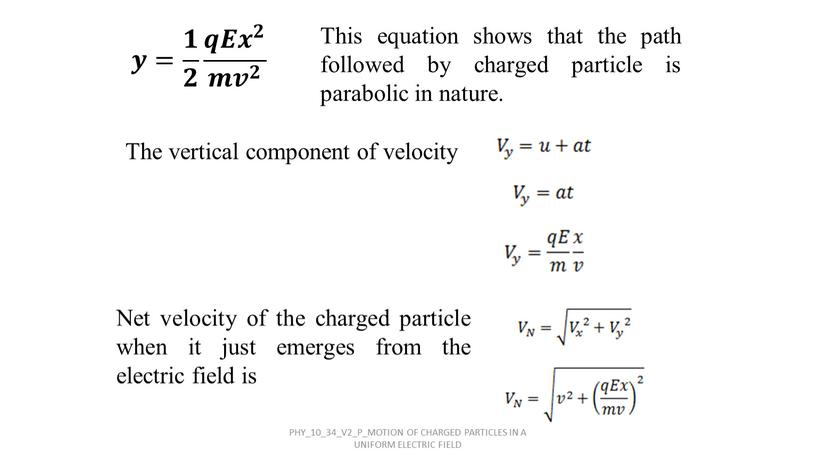𝒚= 𝟏 𝟐 𝒒𝑬 𝒙 𝟐 𝒎 𝒗 𝟐

This equation shows that the path followed by charged particle is parabolic in nature.

The vertical component of velocity

Net velocity of the charged particle when it just emerges from the electric field is

PHY_10_34_V2_P_MOTION OF CHARGED PARTICLES IN A UNIFORM ELECTRIC FIELD

PHY_10_34_V2_P_MOTION OF CHARGED PARTICLES IN A UNIFORM ELECTRIC FIELD

CATHODE RAY TUBE

PHY_10_34_V2_P_MOTION OF CHARGED PARTICLES IN A UNIFORM ELECTRIC FIELD

Label the parts of the oscilloscope

What is CRT?
For what reason an electron gun is used?
Responsibility of the vertical and horizontal plates…
How do we call the screen on which electrons gives a bright spot

Watch the video (0-1,25 min) AND ANSWER THE QUESTIONS: